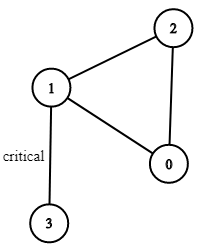## Algorithm

Problem Name: 1192. Critical Connections in a Network

There are `n` servers numbered from `0` to `n - 1` connected by undirected server-to-server `connections` forming a network where `connections[i] = [ai, bi]` represents a connection between servers `ai` and `bi`. Any server can reach other servers directly or indirectly through the network.

A critical connection is a connection that, if removed, will make some servers unable to reach some other server.

Return all critical connections in the network in any order.

Example 1:```Input: n = 4, connections = [[0,1],[1,2],[2,0],[1,3]]
Output: [[1,3]]
Explanation: [[3,1]] is also accepted.
```

Example 2:

```Input: n = 2, connections = [[0,1]]
Output: [[0,1]]
```

Constraints:

• `2 <= n <= 105`
• `n - 1 <= connections.length <= 105`
• `0 <= ai, bi <= n - 1`
• `ai != bi`
• There are no repeated connections.

## Code Examples

### #1 Code Example with Java Programming

```Code - Java Programming```

``````
class Solution {
public List> criticalConnections(int n, List> connections) {
Map> graph = new HashMap<>();
Map rank = new HashMap<>();
Set connectionSet = new HashSet<>();
buildGraph(n, connections, graph, rank, connectionSet);
dfs(0, 0, graph, rank, connectionSet);
List> result = new ArrayList<>();
for (String conn : connectionSet) {
int nodeOne = Integer.parseInt(conn.split("-"));
int nodeTwo = Integer.parseInt(conn.split("-"));
}
return result;
}

private void buildGraph(int n, List> connections, Map> graph, Map rank, Set connectionSet) {
for (int i = 0; i < n; i++) {
graph.put(i, new ArrayList<>());
rank.put(i, null);
}
for (List edge : connections) {
Math.min(edge.get(0), edge.get(1)) + "-" + Math.max(edge.get(0), edge.get(1)));
}
}

private int dfs(int node, int discoveryRank, Map> graph, Map rank, Set connectionSet) {
if (rank.get(node) != null) {
return rank.get(node);
}
rank.put(node, discoveryRank);
int minimumRank = discoveryRank + 1;
for (Integer neighbor : graph.get(node)) {
Integer neighborRank = rank.get(neighbor);
if (neighborRank != null && neighborRank == discoveryRank - 1) {
continue;
}
int recursiveRank = dfs(neighbor, discoveryRank + 1, graph, rank, connectionSet);
if (recursiveRank <= discoveryRank) {
connectionSet.remove(Math.min(node, neighbor) + "-" + Math.max(node, neighbor));
}
minimumRank = Math.min(minimumRank, recursiveRank);
}
return minimumRank;
}
}
``````
Copy The Code &

Input

cmd
n = 4, connections = [[0,1],[1,2],[2,0],[1,3]]

Output

cmd
[[1,3]]

### #2 Code Example with Javascript Programming

```Code - Javascript Programming```

``````
const criticalConnections = function(n, connections) {
const g = [],
low = Array(n),
res = []
low.fill(0)
for (let con of connections) {
g[con] = g[con] || []
g[con] = g[con] || []
g[con].push(con)
g[con].push(con)
}
const dfs = function(cur, v, p) {
let dfn = cur
low[v] = cur
for (let i of g[v]) {
if (i != p) {
if (low[i] == 0) {
cur++
dfs(cur, i, v)
if (low[i] > dfn) {
res.push([i, v])
}
}
low[v] = Math.min(low[v], low[i])
}
}
}
dfs(1, 0, -1)
return res
}
``````
Copy The Code &

Input

cmd
n = 4, connections = [[0,1],[1,2],[2,0],[1,3]]

Output

cmd
[[1,3]]

### #3 Code Example with C# Programming

```Code - C# Programming```

``````
using System;
using System.Collections.Generic;
using System.Linq;

namespace LeetCode
{
public class _1192_CriticalConnectionsInANetwork
{
public IList> CriticalConnections(int n, IList> connections)
{
var graph = new Dictionary>();
var edges = new HashSet<(int, int)>();
foreach (var connection in connections)
{
if (!graph.ContainsKey(connection))
graph[connection] = new List();
if (!graph.ContainsKey(connection))
graph[connection] = new List();

}

var ranks = new int[n];
for (int i = 0; i < n; i++)
ranks[i] = -2;

DFS(0, 0, graph, edges, ranks, n);

return edges.Select(a => new int { a.Item1, a.Item2 }).ToList>();
}

private int DFS(int node, int depth, Dictionary> graph, HashSet<(int, int)> edges, int[] ranks, int n)
{
if (ranks[node] >= 0) return ranks[node];

ranks[node] = depth;
var minStartPoint = n;
foreach (var neighbor in graph[node])
{
if (ranks[neighbor] == depth - 1) continue;
var startPoint = DFS(neighbor, depth + 1, graph, edges, ranks, n);
if (startPoint <= depth)
{
if (edges.Contains((node, neighbor)))
edges.Remove((node, neighbor));
else if (edges.Contains((neighbor, node)))
edges.Remove((neighbor, node));
}
minStartPoint = Math.Min(minStartPoint, startPoint);
}
return minStartPoint;
}
}
}
``````
Copy The Code &

Input

cmd
n = 2, connections = [[0,1]]

Output

cmd
[[0,1]]